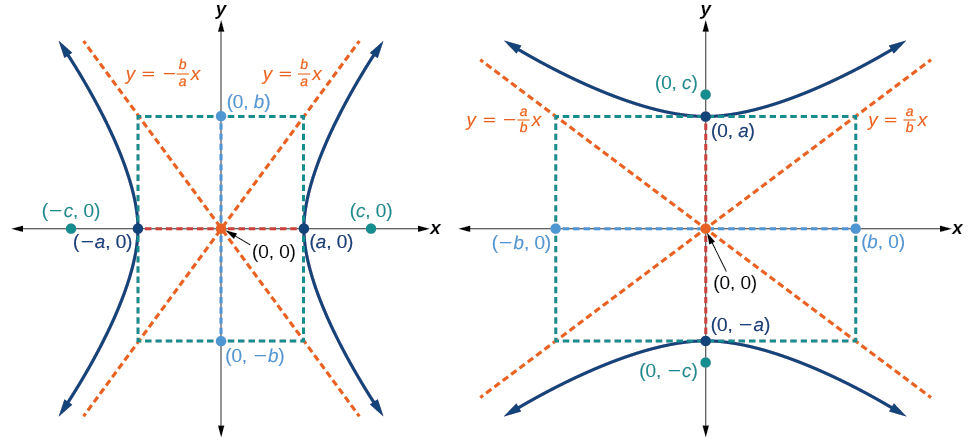# 10.2 The hyperbola  (Page 3/13)

 Page 3 / 13(a) Horizontal hyperbola with center   ( 0 , 0 )   (b) Vertical hyperbola with center   ( 0 , 0 )

Given the equation of a hyperbola in standard form, locate its vertices and foci.

1. Determine whether the transverse axis lies on the x - or y -axis. Notice that $\text{\hspace{0.17em}}{a}^{2}\text{\hspace{0.17em}}$ is always under the variable with the positive coefficient. So, if you set the other variable equal to zero, you can easily find the intercepts. In the case where the hyperbola is centered at the origin, the intercepts coincide with the vertices.
1. If the equation has the form $\text{\hspace{0.17em}}\frac{{x}^{2}}{{a}^{2}}-\frac{{y}^{2}}{{b}^{2}}=1,$ then the transverse axis lies on the x -axis. The vertices are located at $\text{\hspace{0.17em}}\left(±a,0\right),$ and the foci are located at $\text{\hspace{0.17em}}\left(±c,0\right).$
2. If the equation has the form $\text{\hspace{0.17em}}\frac{{y}^{2}}{{a}^{2}}-\frac{{x}^{2}}{{b}^{2}}=1,$ then the transverse axis lies on the y -axis. The vertices are located at $\text{\hspace{0.17em}}\left(0,±a\right),$ and the foci are located at $\text{\hspace{0.17em}}\left(0,±c\right).$
2. Solve for $\text{\hspace{0.17em}}a\text{\hspace{0.17em}}$ using the equation $\text{\hspace{0.17em}}a=\sqrt{{a}^{2}}.$
3. Solve for $\text{\hspace{0.17em}}c\text{\hspace{0.17em}}$ using the equation $\text{\hspace{0.17em}}c=\sqrt{{a}^{2}+{b}^{2}}.$

## Locating a hyperbola’s vertices and foci

Identify the vertices and foci of the hyperbola    with equation $\text{\hspace{0.17em}}\frac{{y}^{2}}{49}-\frac{{x}^{2}}{32}=1.$

The equation has the form $\text{\hspace{0.17em}}\frac{{y}^{2}}{{a}^{2}}-\frac{{x}^{2}}{{b}^{2}}=1,$ so the transverse axis lies on the y -axis. The hyperbola is centered at the origin, so the vertices serve as the y -intercepts of the graph. To find the vertices, set $\text{\hspace{0.17em}}x=0,$ and solve for $\text{\hspace{0.17em}}y.$

$\begin{array}{l}\text{\hspace{0.17em}}\text{\hspace{0.17em}}\text{\hspace{0.17em}}1=\frac{{y}^{2}}{49}-\frac{{x}^{2}}{32}\hfill \\ \text{\hspace{0.17em}}\text{\hspace{0.17em}}\text{\hspace{0.17em}}1=\frac{{y}^{2}}{49}-\frac{{0}^{2}}{32}\hfill \\ \text{\hspace{0.17em}}\text{\hspace{0.17em}}\text{\hspace{0.17em}}1=\frac{{y}^{2}}{49}\hfill \\ {y}^{2}=49\hfill \\ \text{\hspace{0.17em}}\text{\hspace{0.17em}}\text{\hspace{0.17em}}y=±\sqrt{49}=±7\hfill \end{array}$

The foci are located at $\text{\hspace{0.17em}}\left(0,±c\right).\text{\hspace{0.17em}}$ Solving for $\text{\hspace{0.17em}}c,$

$c=\sqrt{{a}^{2}+{b}^{2}}=\sqrt{49+32}=\sqrt{81}=9$

Therefore, the vertices are located at $\text{\hspace{0.17em}}\left(0,±7\right),$ and the foci are located at $\text{\hspace{0.17em}}\left(0,9\right).$

Identify the vertices and foci of the hyperbola with equation $\text{\hspace{0.17em}}\frac{{x}^{2}}{9}-\frac{{y}^{2}}{25}=1.$

Vertices: $\text{\hspace{0.17em}}\left(±3,0\right);\text{\hspace{0.17em}}$ Foci: $\text{\hspace{0.17em}}\left(±\sqrt{34},0\right)$

## Writing equations of hyperbolas in standard form

Just as with ellipses, writing the equation for a hyperbola in standard form allows us to calculate the key features: its center, vertices, co-vertices, foci, asymptotes, and the lengths and positions of the transverse and conjugate axes. Conversely, an equation for a hyperbola can be found given its key features. We begin by finding standard equations for hyperbolas centered at the origin. Then we will turn our attention to finding standard equations for hyperbolas centered at some point other than the origin.

## Hyperbolas centered at the origin

Reviewing the standard forms given for hyperbolas centered at $\text{\hspace{0.17em}}\left(0,0\right),$ we see that the vertices, co-vertices, and foci are related by the equation $\text{\hspace{0.17em}}{c}^{2}={a}^{2}+{b}^{2}.\text{\hspace{0.17em}}$ Note that this equation can also be rewritten as $\text{\hspace{0.17em}}{b}^{2}={c}^{2}-{a}^{2}.\text{\hspace{0.17em}}$ This relationship is used to write the equation for a hyperbola when given the coordinates of its foci and vertices.

Given the vertices and foci of a hyperbola centered at $\text{\hspace{0.17em}}\left(0,\text{\hspace{0.17em}}\text{0}\right),$ write its equation in standard form.

1. Determine whether the transverse axis lies on the x - or y -axis.
1. If the given coordinates of the vertices and foci have the form $\text{\hspace{0.17em}}\left(±a,0\right)\text{\hspace{0.17em}}$ and $\text{\hspace{0.17em}}\left(±c,0\right),\text{\hspace{0.17em}}$ respectively, then the transverse axis is the x -axis. Use the standard form $\text{\hspace{0.17em}}\frac{{x}^{2}}{{a}^{2}}-\frac{{y}^{2}}{{b}^{2}}=1.$
2. If the given coordinates of the vertices and foci have the form $\text{\hspace{0.17em}}\left(0,±a\right)\text{\hspace{0.17em}}$ and $\text{\hspace{0.17em}}\left(0,±c\right),\text{\hspace{0.17em}}$ respectively, then the transverse axis is the y -axis. Use the standard form $\text{\hspace{0.17em}}\frac{{y}^{2}}{{a}^{2}}-\frac{{x}^{2}}{{b}^{2}}=1.$
2. Find $\text{\hspace{0.17em}}{b}^{2}\text{\hspace{0.17em}}$ using the equation $\text{\hspace{0.17em}}{b}^{2}={c}^{2}-{a}^{2}.$
3. Substitute the values for $\text{\hspace{0.17em}}{a}^{2}\text{\hspace{0.17em}}$ and $\text{\hspace{0.17em}}{b}^{2}\text{\hspace{0.17em}}$ into the standard form of the equation determined in Step 1.

what is set?
a colony of bacteria is growing exponentially doubling in size every 100 minutes. how much minutes will it take for the colony of bacteria to triple in size
I got 300 minutes. is it right?
Patience
no. should be about 150 minutes.
Jason
It should be 158.5 minutes.
Mr
ok, thanks
Patience
100•3=300 300=50•2^x 6=2^x x=log_2(6) =2.5849625 so, 300=50•2^2.5849625 and, so, the # of bacteria will double every (100•2.5849625) = 258.49625 minutes
Thomas
what is the importance knowing the graph of circular functions?
can get some help basic precalculus
What do you need help with?
Andrew
how to convert general to standard form with not perfect trinomial
can get some help inverse function
ismail
Rectangle coordinate
how to find for x
it depends on the equation
Robert
yeah, it does. why do we attempt to gain all of them one side or the other?
Melissa
whats a domain
The domain of a function is the set of all input on which the function is defined. For example all real numbers are the Domain of any Polynomial function.
Spiro
Spiro; thanks for putting it out there like that, 😁
Melissa
foci (–7,–17) and (–7,17), the absolute value of the differenceof the distances of any point from the foci is 24.
difference between calculus and pre calculus?
give me an example of a problem so that I can practice answering
x³+y³+z³=42
Robert
dont forget the cube in each variable ;)
Robert
of she solves that, well ... then she has a lot of computational force under her command ....
Walter
what is a function?
I want to learn about the law of exponent
explain this
what is functions?
A mathematical relation such that every input has only one out.
Spiro
yes..it is a relationo of orders pairs of sets one or more input that leads to a exactly one output.
Mubita
Is a rule that assigns to each element X in a set A exactly one element, called F(x), in a set B.
RichieRich

#### Get Jobilize Job Search Mobile App in your pocket Now!ByByBy OpenStaxBy OpenStaxBy Saylor FoundationBy Rohini AjayBy OpenStaxBy Edgar DelgadoBy OpenStaxBy OpenStaxBy Stephen VoronBy OpenStax